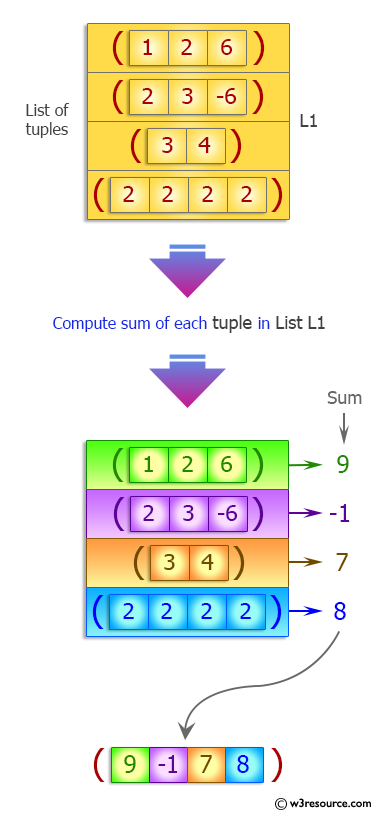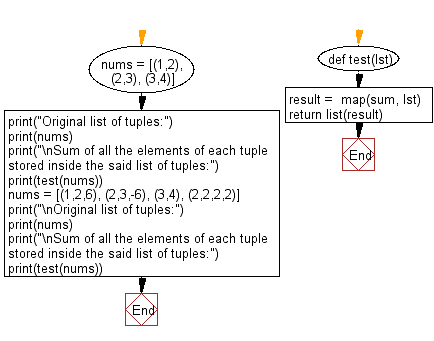﻿ Python: Sum of all the elements of each tuple stored inside a list of tuples - w3resource# Python Exercise: Sum of all the elements of each tuple stored inside a list of tuples

## Python tuple: Exercise-32 with Solution

Write a Python program to compute the sum of all the elements of each tuple stored inside a list of tuples.

Sample Solution:-

Python Code:

``````def test(lst):
result =  map(sum, lst)
return list(result)

nums = [(1,2), (2,3), (3,4)]
print("Original list of tuples:")
print(nums)
print("\nSum of all the elements of each tuple stored inside the said list of tuples:")
print(test(nums))

nums = [(1,2,6), (2,3,-6), (3,4), (2,2,2,2)]
print("\nOriginal list of tuples:")
print(nums)
print("\nSum of all the elements of each tuple stored inside the said list of tuples:")
print(test(nums))
```
```

Sample Output:

```Original list of tuples:
[(1, 2), (2, 3), (3, 4)]

Sum of all the elements of each tuple stored inside the said list of tuples:
[3, 5, 7]

Original list of tuples:
[(1, 2, 6), (2, 3, -6), (3, 4), (2, 2, 2, 2)]

Sum of all the elements of each tuple stored inside the said list of tuples:
[9, -1, 7, 8]
```

Pictorial Presentation:Flowchart:## Visualize Python code execution:

The following tool visualize what the computer is doing step-by-step as it executes the said program:

Python Code Editor:

Have another way to solve this solution? Contribute your code (and comments) through Disqus.

What is the difficulty level of this exercise?

Test your Programming skills with w3resource's quiz.

﻿

## Python: Tips of the Day

Decapitalizes the first letter of a string:

Example:

```def tips_decapitalize(s, upper_rest=False):
return s[:1].lower() + (s[1:].upper() if upper_rest else s[1:])
print(tips_decapitalize('PythonTips'))
print(tips_decapitalize('PythonTips', True))
```

Output:

```pythonTips
pYTHONTIPS
```